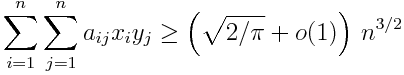# How many lights can you turn on?

Suppose you have a large n × n grid of lights, some turned on and some turned off. Along the side of each row is a switch that can toggle the lights in that row, turning on lights that were originally off and vice versa. There are similar switches along the top that can toggle the lights in each column. How many lights can you turn on?

The answer depends on the initial configuration. If they’re all on initially, for example, then you don’t need to do anything! But in the worst case, how well can you do?

Let aij be +1 or -1 depending on whether the light in the ith row and jth column is initially turned on or off. Let xi be +1 or -1 depending on whether the lights in the ith row are toggled, and let yj be +1 or -1 depending on whether the lights in the jth column are toggled. It is always possible to choose the values of xi and yj so thatHere o(1) means a term that goes to zero as n increases. More on this notation here.

A light that is turned on contributes a +1 to the sum and a light that is turned off contributes a -1, so the sum tells us # lights on – # lights off. Of course # lights on + # lights off = n2, so the number of lights on is(Note that o(1)/2 = o(1): half of an expression going to zero is still an expression going to zero.)

The proof takes about a page in The Probabilistic Method. The idea is to pick the yj randomly and then select the xi to maximize the sum. The lower bound comes from the expected value over the choices of the y‘s. Since the lower bound is the expected value, there must be some choice of y‘s that exceed or at least meet that value.

The theme of The Probabilistic Method is using probability to prove theorems that have nothing to do with probability. For example, sometimes the easiest way to prove an object with a certain property exists is to prove that the probability of selecting such an object at random is positive. As one of my professors used to say, some things are so hard to find that the best way to find them is to look randomly. Sometimes the thing you’re looking for has high probability, particularly in a limit, but your intuition is drawn to low probability exceptions.

## 4 thoughts on “How many lights can you turn on?”

1. Should that be a plus in the last expression? How can the answer be less than n^2 / 2?

2. Yes, should be +. I’ve updated it. Thanks.

3. Interestingly, the initial problem you posed is a particular case of the NP-hard optimization problem MAX-3-LIN-2: given a set of linear equations over F_2 (field with two elements) each involving at most 3 variables, what is the maximum number of those equations that can be simultaneously satisfied? The proof you mention more or less gives a randomized algorithm with approximation ratio of 1/2, since the leading term in your expression is n^2 / 2. (I say “more or less” because to guarantee something sufficiently close to the expectation one also needs concentration bounds.)

Hastad showed that approximating MAX-3-LIN-2 to any constant factor greater than 1/2 implies P=NP.

The phenomenon here is oft-repeated: for many NP-hard optimization problems there is often a simple randomized (or even deterministic) approximation algorithm that approximates the optimum within some factor and improving that factor at all implies P=NP.

4. Dave Tate

Sometimes the thing you’re looking for has high probability, particularly in a limit, but your intuition is drawn to low probability exceptions.

My favorite example of this has to do with normal numbers. The probability of choosing a non-normal number at random from a real interval is zero, but any number you can write down is non-normal, and (almost?) every real number you have ever used is non-normal. Even worse, only a handful of specific real numbers are known to be normal.